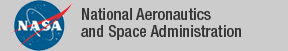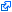### Developments and Contributions

See the dependency graph of all the contributions in the NASA PVS Library NASA PVS Library 6.0.9 (11/10/14).

Development Description Contributors
ACCoRD (web) Framework for the analysis of air traffic conflict detection and resolution algorithms. AN, CM, GD, GH, JM, RB
algebra Groups, monoids, rings, etc. DL, RB
analysis Real analysis, limits, continuity, derivatives, integrals. AN, BD, RB
Bernstein (web) Formalization of multivariate Bernstein polynomials. AN, CM
complex Complex numbers.DL
complex_alt Alternative formalization of complex numbers. DL
complex_integration Complex integration.DL
co_structures Sequences of countable length defined as coalgebra datatypes.JJ
digraphs Directed graphs: circuits, maximal subtrees, paths, DAGs. AA, JS, KR, RB
exact_real_arith Exact real arithmetic including trig functions.DL
extended_nnreal Extended non-negative reals.DL
fault_tolerance Fault tolerance protocols. AG, JM, LP, PM
float Floating point numbers and arithmetic. PM, SB
graphs Graph theory: connectedness, walks, trees, Menger's Theorem. RB, JS
groups Group theory.ALG
ints Integer division, gcd, mod, prime factorization, min, max. AG, BD, PM, RB
interval_arith (web) Interval arithmetic and numerical approximations. Includes automated strategies `numerical` for computing numerical approximations and `interval` for checking satisfiability and validity of simply quantified real-valued formulas. This development includes a formalization of Allen interval temporal logic. AN, CM, DS
lebesgue Lebesgue integral with connection to Riemann Integral.DL
linear_algebra Linear algebra.HH, GP, SO
lnexp Logarithm, exponential and hyperbolic functions. DL, RB
lnexp_fnd Foundational definitions of logarithm, exponential and hyperbolic functions. AN, DL, RB
measure_integration Sigma algebras, measures, Fubini-Tonelli Lemmas.DL
metric_space Domains with a distance metric, continuity and uniform continuity.DL
numbers Elementary number theory. AG, AN, RB
orders Abstract orders, lattices, fix points. AG, BD, JJ
power Generalized Power function (without ln/exp). DL
probability Probability theory.DL
reals Summations, sup, inf, sqrt over the reals, absolute value, etc. AN, BDV, CM, PM, RB, AI
scott Scott topology.DL
series Power series, comparison test, ratio test, Taylor's theorem. AN, DL, RB
sets_aux Powersets, orders, cardinality over infinite sets. Includes functional and relational facts based on Axiom of Choice and refinement relations based on equivalence relations. BD, DL, JJ, DS
sigma_set Summations over countably infinite sets. DL
structures Bounded arrays, finite sequences, bags, and several other structures. CM, DG, KR, LP, RB
Sturm (web) Formalization of Sturm's theorem for univariate polynomials. Includes strategies `sturm` and `mono-poly` for automatically proving univariate polynomial relations over a real interval. AN, CM, AD
Tarski (web) Formalization of Tarski's theorem for univariate polynomials. Includes strategy `tarski` for automatically proving systems of univaraite polynomial relations on the real line. AN, CM, AD
topology Continuity, homeomorphisms, connected and compact spaces, Borel sets/functions.DL
trig Trigonometry: definitions, identities, approximations. CM, BDV, DL, GD, HG, RB, VC, AN, JVS
TRS Term rewrite systems and Robinson unification algorithm.AA, ALG, AR, MA
TU_games Cooperative TU-games.EM
vect_analysis Limits, continuity, and derivatives of vector functions. AN, CM, RB
vectors 2-D, 3-D, 4-D, and n-dimensional vectors. AN, BDV, CM, HG, PM, RB
while Semantics for the programming language "while".DL

### Major Theorems in the NASA PVS Library

Theorem Theory PVS Name Author
Cauchy-Schwarz Inequality `vectors@vectors``cauchy_schwartz` RB
Derivative of a Power Series `series@power_series_deriv``powerseries_deriv` RB
Fundamental Theorem of Arithmetic `numbers@unique_factorization` `Fundamental_Theorem_Arithmetic` RB
Fundamental Theorem of Calculus `analysis@fundamental_theorem` `fundamental` RB
Fundamental Theorem of Interval Arithmetic `interval_arith@interval_expr` `Eval_fundamental` CM, AN
Inclusion Theorem of Interval Arithmetic `interval_arith@interval_expr` `Eval_inclusion` CM, AN
Infinitude of Primes `numbers@infinite_primes``primes_infinite` RB
Integral of a Power Series `series@power_series_integ``integral_powerseries` RB
Intermediate Value Theorem `analysis@continuity_interval``intermeditate_value1` BD
Law of Cosines `trig_fnd@law_cosines``Law_Cosines` CM
Mean Value Theorem `analysis@derivative_props``mean_value` BD
Matel's Theorem `graphs@mantel``Mantel` AD
Menger's Theorem `graphs@menger``hard_menger` JS
Order of a Subgroup `algebra@finite_group``lagrange` DL
Pythagorean Property - Sine and Cosine `trig_fnd@trig_basic``sin2_cos2` DL
Ramsey's Theorem `graphs@ramsey``ramseys_theorem` NS
Sum of a Geometric Series `series@series` `geometric_sum ` RB
Taylor's Theorem `analysis@taylors` `Taylors` RB
Trig Identities: Sum and Diff of Two Angles `trig_fnd@trig_basic` `sin_plus`, ... DL
Trig Identities: Double Angle Formulas `trig_fnd@rig_basic` `sin2a, cos2s` DL
Schroeder-Berstein Theorem `orders@set_antinsymmetric` `inj_inj_bij` JJ
Denumerability of the Rational Numbers `sets_aux@countable_set` `countable_rat` JJ
Heine Theorem and Multiary Variants `analysis@uniform_continuity` `Heine`, `multiary_Heine` AN
Fubini-Tonelli Lemmas `measure_integration@fubini_tonelli` `fubini_tonelli_*` DL
Knuth-Bendix Critical Pair Theorem `TRS@critical_pairs``CP_Theorem` ALG, MA
Church-Rosser Theorem `TRS@results_confluence``CR_iff_Confluent` ALG, MA
Newman Lemma `TRS@newman_yokouchi``Newman_lemma` ALG, MA
Yokouchi Lemma `TRS@newman_yokouchi``Yokouchi_lemma` ALG, MA
Robinson Unification `TRS@robinsonunification``completeness_robinson_unification_algorithm` AA, MA
Confluence of Orthogonal TRSs `TRS@orthogonality``Orthogonal_implies_confluent` AR, MA
Sturm's Theorem `Sturm@sturm``sturm` AN
Tarski's Theorem `Tarski@sturmtarski``sturm_tarski_unbounded` AN, AD

### Contributors

AA Andreia Avelar Borges, University of Brasilia, Brazil Aaron Dutle, NASA, USA Alfons Geser, HTWK Leipzig, Germany Amy Isvik, Wartburg College, USA André Galdino, Federal University of Goiás, Brazil Anthony Narkawicz, NASA, USA Ana Cristina Rocha Oliveira, University of Brasilia, Brazil Bruno Dutertre, SRI, USA Ben Di Vito, NASA, USA César Muñoz, NASA, USA David Griffioen,CWI, The Netherlands David Lester, Manchester University, UK Dragan Stosic, Ireland Érik Martin-Dorel, U. Montpellier 2 & U. of Perpignan (formerly), France Heber Herencia-Zapana, NIA, USA Jerry James, Utah State University (formerly), USA Jeff Maddalon, NASA, USA Jon Sjogren, Department of Defense, USA John Siratt, University of Arkansas at Little Rock, USA Kristin Rozier, NASA, USA Gilles Dowek, INRIA, France Geroge Hagen, NASA, USA Gilberto Perez, University of La Coruña, Spain Hanne Gottliebsen, NIA (formerly), USA Lee Pike, Galois, USA Mauricio Ayala-Rincón, University of Brasilia, Brazil Natarajan Shankar, SRI, USA Paul Miner, NASA, USA Ricky Butler, NASA, USA Silvie Boldo, INRIA, France Sam Owre, SRI, USA Víctor Carreño, NASA (formerly), USA

If we have incorrectly attributed a PVS development, please let us know.

The tagidentifies links that are outside the NASA domain